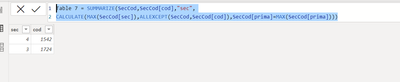cancel
Showing results for
Did you mean:Helper I

## values from Order_By column within Summar table

Hi,

I used summarize in the (sales) table, and grouped by columns [Cod] and [Sec], being the summary expressión "prima" a simple sum. The virtual summarized table would look like this.

 Cod Sec prima 1542 1 \$1.425,75 1542 4 \$2.722,30 1724 3 \$2.524,11 1724 8 \$241,00

Now, from this virtual summarized table,  I need the get the values of [Sec] colum for the max (prima) for each [Cod], that woul be "SecOfMaxPrima". The returned value would a table containing at least  columns [Cod] and [SecOfMaxPrima]

In this example, returned values should be

 Cod Sec 1542 4 1724 3

I failed at using lookupvalue for this, since it doesn´t recognize the virtual summary table. I failed also at summarizing the summarized table (can you do that?)

1 ACCEPTED SOLUTIONCommunity Support

Hi @DavidGolden,

Did you mean the sample table that you shared has been summarized already? If that is the case, you can try to use the following formulas to create a new table if it is suitable for your requirement:

``````NewTable =
VAR summary =
SUMMARIZE ( 'Table', [Cod], [Sec], "s_prima", SUM ( 'Table'[prima] ) )
VAR mPrima =
GROUPBY ( summary, [Cod], "prima", MAXX ( CURRENTGROUP (), [s_prima] ) )
RETURN
FILTER ( summary, [s_prima] IN SELECTCOLUMNS ( mPrima, "prima", [prima] ) )``````

Regards,

Xiaoxin Sheng

Community Support Team _ Xiaoxin
If this post helps, please consider accept as solution to help other members find it more quickly.
4 REPLIES 4Super User

Hi,

Here is one way to do this:

Table 7 = SUMMARIZE(SecCod,SecCod[cod],"sec",
CALCULATE(MAX(SecCod[sec]),ALLEXCEPT(SecCod,SecCod[cod]),SecCod[prima]=MAX(SecCod[prima])))
Example:I hope this post helps to solve your issue and if it does consider accepting it as a solution and giving the post a thumbs up!

Proud to be a Super User!Helper I

Thnaks @ValtteriN , can I do that within a virtual table taht has been already summarized?

I mean, the 'SecCod' table you suggest is actually a returned table from summarize function, I tryed your suggestion, but it didnt worked. Am I missing something?Community Support

Hi @DavidGolden,

Did you mean the sample table that you shared has been summarized already? If that is the case, you can try to use the following formulas to create a new table if it is suitable for your requirement:

``````NewTable =
VAR summary =
SUMMARIZE ( 'Table', [Cod], [Sec], "s_prima", SUM ( 'Table'[prima] ) )
VAR mPrima =
GROUPBY ( summary, [Cod], "prima", MAXX ( CURRENTGROUP (), [s_prima] ) )
RETURN
FILTER ( summary, [s_prima] IN SELECTCOLUMNS ( mPrima, "prima", [prima] ) )``````

Regards,

Xiaoxin Sheng

Community Support Team _ Xiaoxin
If this post helps, please consider accept as solution to help other members find it more quickly.Super User

Hi,

You can place your previous DAX within a variable and summarize it. Do you want to have your end result as a measure or as a table? I am not sure I understood your goal correctly.

Proud to be a Super User!